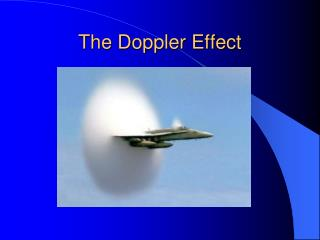DownloadDownload PresentationThe Doppler Effect

# The Doppler Effect

Download Presentation## The Doppler Effect

- - - - - - - - - - - - - - - - - - - - - - - - - - - E N D - - - - - - - - - - - - - - - - - - - - - - - - - - -
##### Presentation Transcript

1. The Doppler Effect

2. The Doppler Effect The apparent frequency of a sound changes due to the relative movement of the source and/or observer http://www.falstad.com/ripple YouTube - Fire Engine siren demonstrates the Doppler Effect

3. Calculating the change in frequency Imagine a car emitting a continuous sound, speed = v, frequency = f Sound source frequency f Observer

4. Stationary Source In a time Δt, the observer will receive fΔt waves if the source is at rest These waves will occupy a distance vΔt (distance = speed x time) Number of waves per second Time in seconds Sound source frequency f Observer

5. Moving Source If the source is now moving with speed us towards the observer The same number of waves will have been emitted in time Δt but this time the waves will occupy a distance of vΔt - usΔt us Distance moved by source Distance moved by waves

6. Moving Source The “new” wavelength λn = distance occupied by waves number of waves λn =vΔt – usΔt fΔt λn = (v – us) f So (v – us) = λn = v f fn where fn is the frequency heard by the observer So fn = vf (v – us) If the source is moving away from the observer, fn = vf/ (v + us) us YouTube - Doppler Effect Demonstration (Using a Little Boy)Related Videos

7. Moving Observer Moving Observer Moving Observer Imagine the observer moving towards the sound source with velocity = vo Imagine the observer moving towards the sound source with velocity = vo Imagine the observer moving towards the sound source with velocity = uo (Sound waves moving with velocity v) The speed of the sound waves as measured by the observer will be v + uo v vo uo

8. Moving Observer We therefore have a situation for the observer where v + uo = fnλ Remembering that for the original wave λ = v/f v + uo = fnλ = fn x v/f From which fn = (1 + uo/v)f If the observer is moving away from the source, fn = (1 - uo/v)f

9. Other effects • Don’t forget that the loudness of the sound will also change as a sound source passes an observer

10. Other effects • The sonic boom YouTube - Sonic Boom - Extreme Close Fly ByRelated Videos

11. Sonic boom • When an airplane travels at a speed faster than sound, density waves of sound emitted by the plane cannot precede the plane, and so accumulate in a cone behind the plane. When this shock wave passes, a listener hears all at once the sound emitted over a longer period: a sonic boom. As a plane accelerates to just break the sound barrier, however, an unusual cloud might form. The origin of this cloud is still debated. A leading theory is that a drop in air pressure at the plane described by the Prandtl-Glauert Singularity occurs so that moist air condenses there to form water droplets.

12. Doppler effect in light • Δf = (v/c)f when v << c

13. Uses of the Doppler effect Measuring the speed of moving objects (like cars or baseballs Measuring the speed of flow of blood cells in an artery

14. Example A sound wave of frequency 300 Hz is emitted towards an approaching car. The wave is reflected from the car and is then received back at the emitter with a frequency of 315 Hz. What is the speed of the car? (Take the speed of sound to be 340 m.s-1).

15. Example A sound wave of frequency 300 Hz is emitted towards an approaching car. The wave is reflected from the car and is then received back at the emitter with a frequency of 315 Hz. What is the speed of the car? (Take the speed of sound to be 340 m.s-1). The car is approaching the emitter, so the frequency the car receives is given by fn = (1 + uo/v)f = (1 + u/340)300

16. Example A sound wave of frequency 300 Hz is emitted towards an approaching car. The wave is reflected from the car and is then received back at the emitter with a frequency of 315 Hz. What is the speed of the car? (Take the speed of sound to be 340 m.s-1). The car is approaching the emitter, so the frequency the car receives is given by fn = (1 + uo/v)f = (1 + u/340)300 The car now acts as the emitter of a wave of this frequency (fn) and the original emitter will now act as the new (stationary) receiver. Thus the frequency received (315 Hz) is given by f = 315 = vfn = 340 x (1 + u/340)300 (v – us) (340-u) From which we find u = 8.29 m.s-1

17. The End Let’s try some questions, pages 248, 249 Can you CHOOSE 2 easy, one medium and one hard one to do?